# Python的shell不是系统终端

## 举例

### 关于python解释器的问题

#### 问题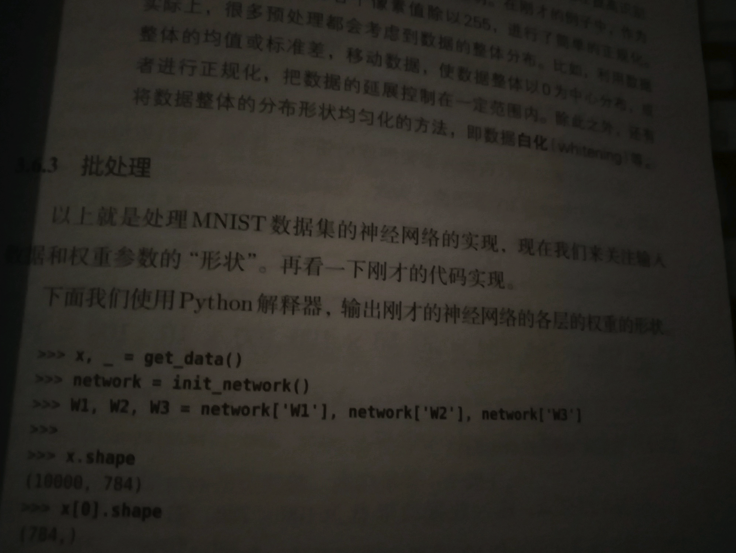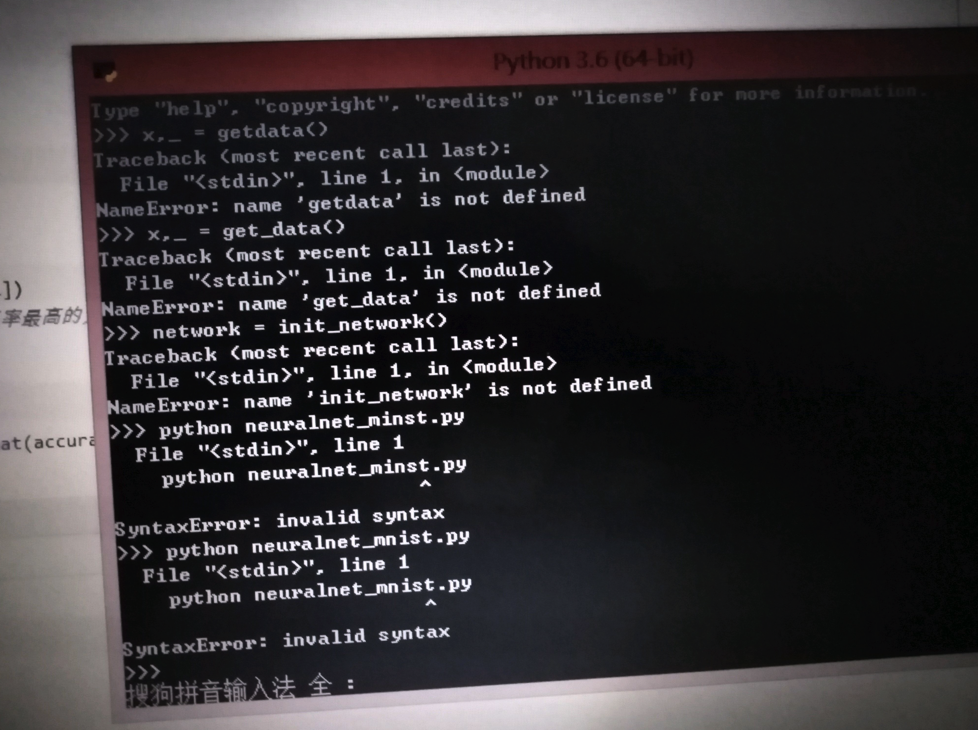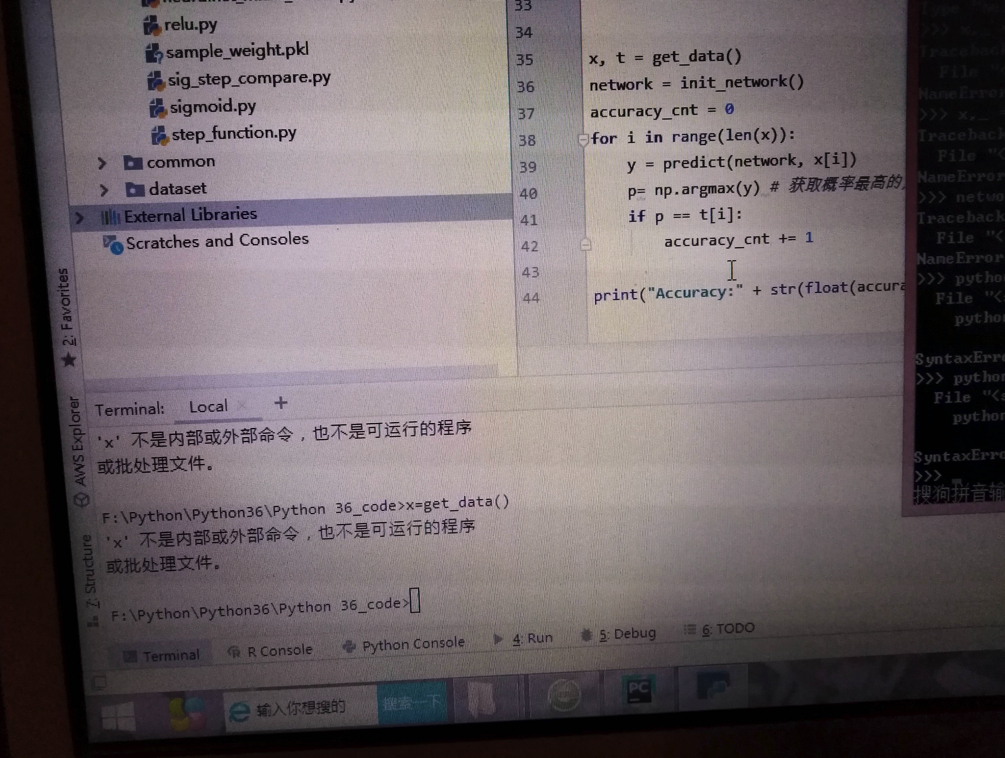#### 解答

• 简答
• 先要搞清楚，书中代码是python shell中运行的
• 其次还要搞清楚，那段get_date()代码，是需要先输入函数定义，才能继续调用执行的
• 都搞清楚后
• 就可以在python shell中输入完整代码，去测试你要的效果了
• 详解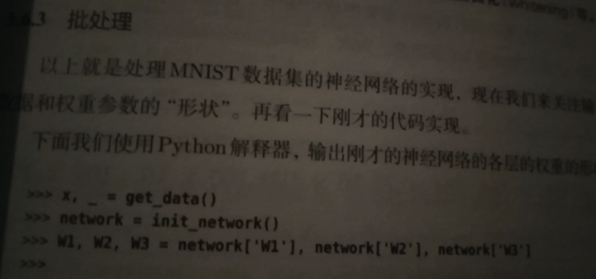``````>>> x, _ = get_data()
>>> network = init_network()
...
``````

``````x, _ get_data()
network = init_network()
...
``````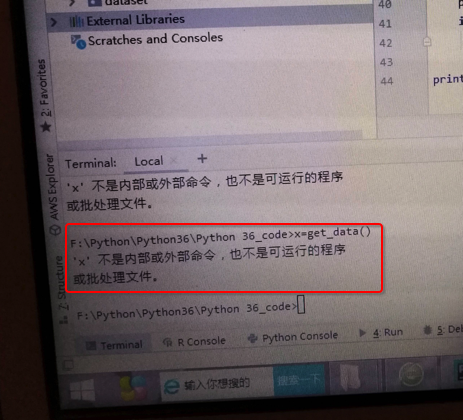``````x=get_data()
‘x’ 不是内部或外部命令，也不是可运行的程序或批处理文件
``````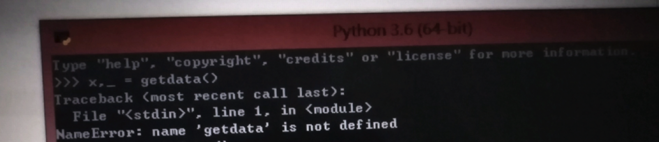• 你不仅（粗心，笔误）把get_data()误写成getdata()
• 还不理解：Python中代码执行的基本逻辑
• get_data()是个函数的调用
• 需要你先把函数实现=函数定义 写出来，或者是导入别的实现了此函数的库
• 然后才能去调用此函数，运行此函数

``````x, _ = get_data()
``````

``````def get_data()
...
return x, y
``````

``````x, _ = get_data()
``````

``````NameError: name ‘getdata’ is not defined
``````

``````x, _ = get_data()
``````

（且确保当前Python环境是OK的，即相关依赖的库已安装等）

python初级教程：入门详解